• 介绍了C++ 十进制转换为二进制的实例代码，有需要的朋友可以参考一下
• 十进制转换为十六进制，也就是 采用 除k取余法 ，直接让10处以16，再对 数据如果小于10和大于10进行处理，代码如下： #include<iostream> #include<string> using namespace std; int main() { ...
十进制转换为十六进制，也就是 采用 除k取余法 ，直接让10处以16，再对 数据如果小于10和大于10进行处理，代码如下：

#include<iostream>
#include<string>
using namespace std;
int main()
{
long a;//输入的数
int y=0;//循环中的余数
string s="";//输出的结果
cin>>a;
if(a==0)//0比较特殊，单独处理
{
cout<<0;
return 0;
}

while(a>0)//大于0的数
{
y=a%16;  //求余
if(y<10)   //小于10的余数
s=char('0'+y)+s;  //利用字符的ascll码在字符串前面拼接
else
s=char('A'-10+y)+s;  //大于9的余数用ABCDE表示
a=a/16;
}
cout<<s;
return 0;
}

十六进制转换为10进制，采取的是 乘以16
#include<iostream>
#include<string>
#include<math.h>
using namespace std;

int main()
{
string s;
int i=0,count,sum;
while(getline(cin,s))
{
int count=s.length();
sum=0;
for(i=count-1;i>=0;i--)//从十六进制个位开始，每位都转换成十进制
{
if(s[i]>='0'&&s[i]<='9')//数字字符的转换
{
sum+=(s[i]-48)*pow(16,count-i-1);
}
else if(s[i]>='A'&&s[i]<='F')//字母字符的转换
{
sum+=(s[i]-55)*pow(16,count-i-1);
}
}
cout<<sum;
}
}  

十进制 转换为 二进制，采取的是 除2取余法，其中把 2 换成其他小于10的数，可以变成相应的 其他进制

#include<iostream>

using namespace std;

int main(){
int a;
cin>>a;
int result=0;
int temp=a;
int j=1;
while(temp){
result=result+j*(temp%2);
temp=temp/2;
j=j*10;
}

cout<<result<<endl;

return 0;
} 

展开全文16进制 进制转换
• ## c++十进制转十六进制

万次阅读 多人点赞 2019-03-11 17:08:08
十六进制转十进制 #include #include int main(){ using namespace std; int i=0,n,m,num; char hex={‘0’,‘1’,‘2’,‘3’,‘4’,‘5’,‘6’,‘7’,‘8’,‘9’,‘A’,‘B’,‘C’,‘D’,‘E’,...
十进制转十六进制
#include<iostream>
#include<cstdlib>
int main(){
using namespace std;
int i=0,n,m,num;
char hex={'0','1','2','3','4','5','6','7','8','9','A','B','C','D','E','F'};
cin>>n;
while(n>0){
num[i++]=n%16;
n=n/16;
}
for(i=i-1;i>=0;i--){
m=num[i];
cout<<hex[m];
}
system("pause");
return 0;
}

展开全文• C++ 十进制转其他进制 开发工具与关键技术：C++、VisualStudio 作者：何任贤 撰写时间：2019年04月10日 在学习C++基础过程中，接触到进制转换，个人想要尝试一下用C++来写一个进制转换， 这篇文章主要内容是十进制...
C++ 十进制转其他进制
开发工具与关键技术：C++、VisualStudio
作者：何任贤
撰写时间：2019年04月10日

在学习C++基础过程中，接触到进制转换，个人想要尝试一下用C++来写一个进制转换， 这篇文章主要内容是十进制转其他进制，现在的进制有十六进制、十进制、八进制、二进制。 我们先来看代码： #include  #include  using namespace std; void main() { int NUM,JZ; int x = 0; int y = 1; int va = 0; string vx = “”; string vr = “”; cout << “请输入一个十进制的数：”; cin >> NUM; cout << “请输入要转换的进制：”; cin >> JZ; if (JZ == 16) { if (NUM >= 16) { for (int i = NUM; i >= 16 * pow(10, x); ) { if (i >= 16 * pow(10, x)) { va = va + 1 * pow(10, y); } i = i - 16 * pow(10, x); if (i<16 * pow(10, x)) { if (i == 10 * pow(10, x)) { vx = “A” + vx; } else if (i == 11 * pow(10, x)) { vx = “B” + vx; } else if (i == 12 * pow(10, x)) { vx = “C” + vx; } else if (i == 13 * pow(10, x)) { vx = “D” + vx; } else if (i == 14 * pow(10, x)) { vx = “E” + vx; } else if (i == 15 * pow(10, x)) { vx = “F” + vx; } else { vx =to_string(i) + vx; } } if (i < 16 * pow(10, x)) { if (va >= 16 * pow(10, y)) { y++; x++; i = va; va = 0; } else { x = vx.size(); va = va / pow(10, x); if (va == 10) { vr = “A”; } else if (va == 11) { vr = “B”; } else if (va == 12) { vr = “C”; } else if (va == 13) { vr = “D”; } else if (va == 14) { vr = “E”; } else if (va == 15) { vr = “F”; } else { vr = to_string(va); } vx = vr + vx; } } } } else { va = NUM; if (va == 10) { vx = “A”; } else if (va == 11) { vx = “B”; } else if (va == 12) { vx = “C”; } else if (va == 13) { vx = “D”; } else if (va == 14) { vx = “E”; } else if (va == 15) { vx = “F”; } else { vx = va; } } } else { for (int i = NUM; i >= JZpow(10, x); ) { if (i >= JZpow(10, x)) { va = va + 1 * pow(10, y); } i = i - JZpow(10, x); if (i<JZpow(10, x)) { va = va + i; vx = to_string(va); } if (i < JZpow(10, x)) { if (va >= JZpow(10, y)) { y++; x++; i = va; va = 0; } } } } cout << “转换之后的数为：” << vx << endl; }  再看一些输出结果： 例1：转八进制和二进制的例子，把它们两个放一起一起讲是因为他们用的是同样的代码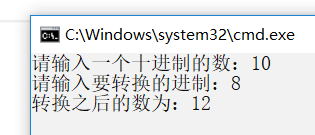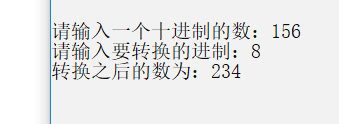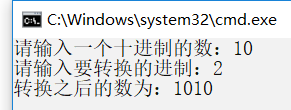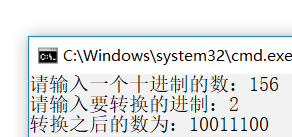这是八进制和二进制的示例，输入的是10和156，输出结果八进制的是12和234，二进制的是1010和10011100，它们的代码主要部分是一个for循环，如上所示NUM代表一个十进制的数，JZ代表要转换的进制，声明两个变量va、vx,当NUM输入的是10，JZ输入的是2 ，该for循环执行每当NUM>=210x时，减去2*10x，x的初始值为0，每当NUM<=210x时加一，va加十，直到NUM等于或小于2时，判断va是否大于等于10的y次方，y的初始值为1，每当NUM<=2*10x时加一，若va大于等于时10的y次方时将va赋值给NUM，继续循环，直到va小于10的y次方，将va赋值给vx然后输出vx。
例2：十六进制和八进制、二进制不同，十六进制有A、B、C、D、E、F等字母，分别代表10、11、12、13、14、15，下面输入和输出结果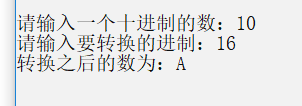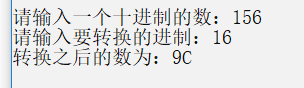输入同样是10和156，输出结果为A和9C，for循环的原理同上，不同的是因为每当十六进制结束一轮for循环时都要加上判断，判断NUM是否为10、11、12、13、14、15，如果是将vx赋值为A、B、C、D、E、F等字母,再进行下轮for循环或结束佛如循环。 以上就是十进制转其他进制的内容，文章到此结束。
展开全文• C++任意进制转换为十进制我们知道任意进制转换为十进制，都是乘以基数的多少次方，然后相加。 废话不多说直接上代码。#include #include using namespace std;int main() { int r,i=0,ans=0; string n; cin>>r>>...
C++任意进制转换为十进制
我们知道任意进制转换为十进制，都是乘以基数的多少次方，然后相加。  废话不多说直接上代码。
#include<iostream>
#include<string>
using namespace std;

int main()
{
int r,i=0,ans=0;
string n;
cin>>r>>n;//R表示进制,N表示要转换的数，ans表示要转换的结果。
while(n.size()!=i)
{
ans*=r;       //我这里是把1看成0，把0看成1来算的。这样比较方便。其实都一样。
ans+=n[i]-'0';
i++;
}
cout<<ans<<endl;
return 0;
} 
十进制转换为任意进制
我们一般的算法都是除以基数，然后倒着取数。大家有没有发现和我们学过的栈是不是很相似。接下来废话不多说，直接上代码。
#include<iostream>
#include<stack>
using namespace std;

int main()
{
int r,n;
stack<int> s;
cin>>n>>r;
while(n)
{
s.push(n%r);
n/=r;
}
while(!s.empty())
{
switch(s.top())
{
case 10:cout<<'A';break;
case 11:cout<<'B';break;
case 12:cout<<'C';break;
case 13:cout<<'D';break;
case 14:cout<<'E';break;
case 15:cout<<'F';break;//这些都是为了能转化为十六进制。
default:cout<<s.top();break;
}
s.pop();
}
return 0;
}

这也是栈的基本应用哦！最后欢迎大家来共同学习，刷题哦！
展开全文string c语言 namespace stack
• Dev C++十进制转m进制 #include int main() {  int a,i,n,m,k,q;  char b;  scanf("%d",&m);//要转换的数  scanf("%d",&n);//转换成几进制  k=m;  if(n>=10)  {  for(i=0;k;)  { ...
• 输入-一个十进制的整数，输出该数的十六进制表示。 输入 输入数据有多组，第一个数表示数据的组数n. 然后是n个十进制数 输出 输出为n行，每行一个对应的十六进制数。其中大于等于10的数字用大写英文字母ABCDEF...
• 1、十进制转换二进制十进制对2整除，得到的余数的倒序即为转换而成的二进制(十进制转换二进制示意图)转换函数#include#include#include#include#includeusing namespace std;void convert_10_to_2(int num){ vector&...
• C++其他进制转十进制 开发工具与关键技术：C++、VisualStudio 作者：何任贤 撰写时间：2019年04月15日 在学习C++基础过程中，接触到进制转换，个人想要尝试一下用C++来写一个进制 转换，这篇文章主要内容是其他...
• 自己写的呀 高手笑过…… 将一个十进制书用二进制格式输出
• 十进制转化为二进制，然后每4位二进制对应1位十六进制，按照相应的数字输出即可。 （用map映射10对应A，11对应B，12对应C.....） #include"iostream" #include"algorithm" #include"...
• 利用这段代码可以实现10进制转换为36进制下的任意进制。 代码的思路很简单，利用一段函数，将每一次取余得到的结果存入数组，最后利用for循环和switch语句将得到的数组反响输出，就得到了转换后的结果。 以下是代码...算法
• 在C中我们可以很简单的实现十进制到十六进制与八进制之间的转换(%x/%X、%o)， 但是却没有直接的方法直接将十进制转换为二进制，这就需要我们自己编写相应的功能函数去实现转化； 本篇文章将介绍两种转化方法： 1&...
• //十进制转换为二进制 #include #include #include #include using namespace std; string s; int main(int argc,char * argv[]) { int n; while(cin>>n) { s=""; for(int a = n; a ;a = a/2
• 如上是将十进制转换1-16进制，并且有可能会输入负数 首先我们先考虑当输入的要转换的数为0，那么不管是任何进制，转换都是0. 然后考虑进制是否小于10，因为大于等于10之后的进制需要使用字符’A’-‘F’. 考虑进制...
• 蓝桥杯练习系统：基础练习 十六进制八进制 C/C++ 十六进制八进制 利用bitset预处理，将十进制转换为对应二进制数
• C++任意进制转换为十进制 我们知道任意进制转换为十进制，都是乘以基数的多少次方，然后相加。 废话不多说直接上代码。 #include&lt;iostream&gt; #include&lt;string&gt; using namespace std; ...
• 今天研究了一下如何将十进制转化为十六进制，并用C++实现了一下，代码可能不是太优化，但还可以用。 在网上一搜才发现之前早有人总结得很全面了，见C++进制转换 本人实现代码如下：#include #include using ...
• 实现读取change.txt内文件，将每行的二进制转为10进制数输出，16进制完成部分。
• ## C++十进制转二进制

千次阅读 2017-12-27 09:40:40
31位的二进制，前面补零 void dec2bin(int num, int bin[]){ for(int i = 31; i&gt;=0; i--){ if(num &amp; (1&lt;&lt;i)) bin[30-i]=1; else bin[30-i]=0; } } 2...
• 十进制转二进制的方法 转化方式原理 不断除以2 ，得到位数， 从下往上读数， 步骤 1、先得到每个余数，放进一个地方，如vector容器 2、再取出得数，逆着取数 代码编写 #include<iostream> #include<vector&...
• //十进制转换为任意进制的源码 #include using namespace std; int main() { long n; int p,c,m=0,s; cout输入要转换的数字："; cin>>n;
• 十进制转换为二进制 较小的二进制 int decToBin(int dec){ int result = 0, temp = dec, j = 1; while(temp){ result = result + j * (temp % 2);...可以推广到十进制转k进制，需要更改的地...
• ## c++十进制转二进制

千次阅读 2018-08-06 11:53:54
void printbinary(const int val) //由 {  unsigned int i = 1; i = i &lt;&lt; (sizeof(i)*8 - 1) //拿到unsigned类型的位数，左移（位数-1）位达到最左边一位为1，然后进for循环判断 ......

# c++十进制转三进制c++ 订阅